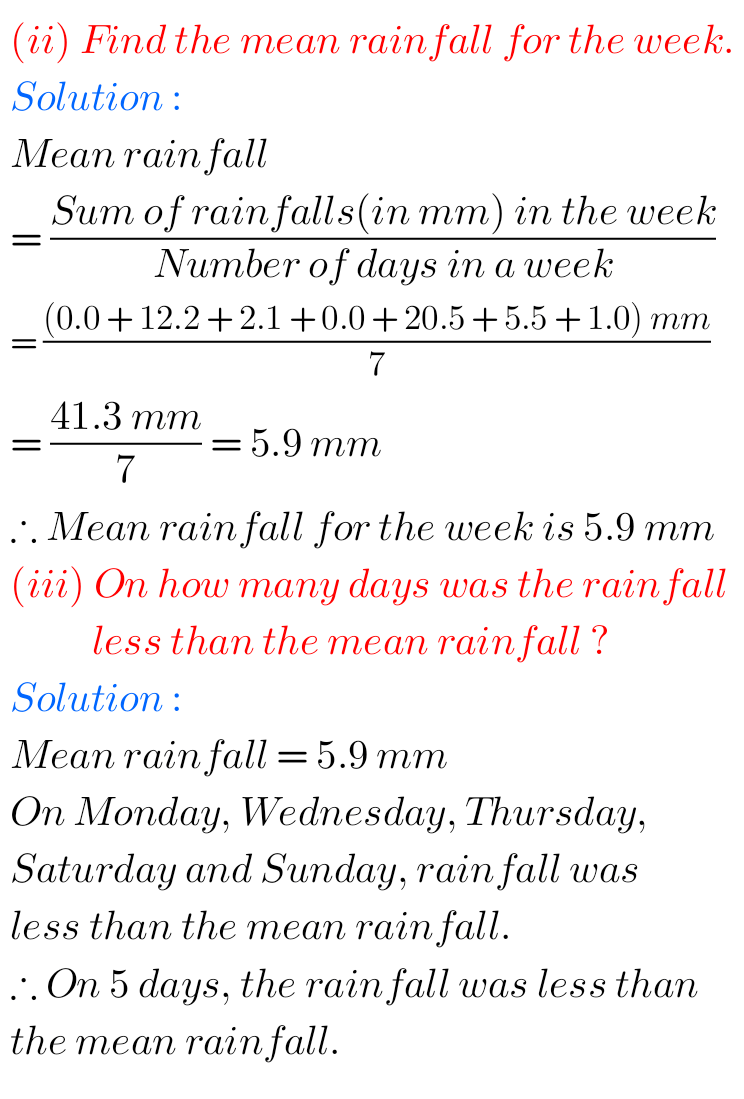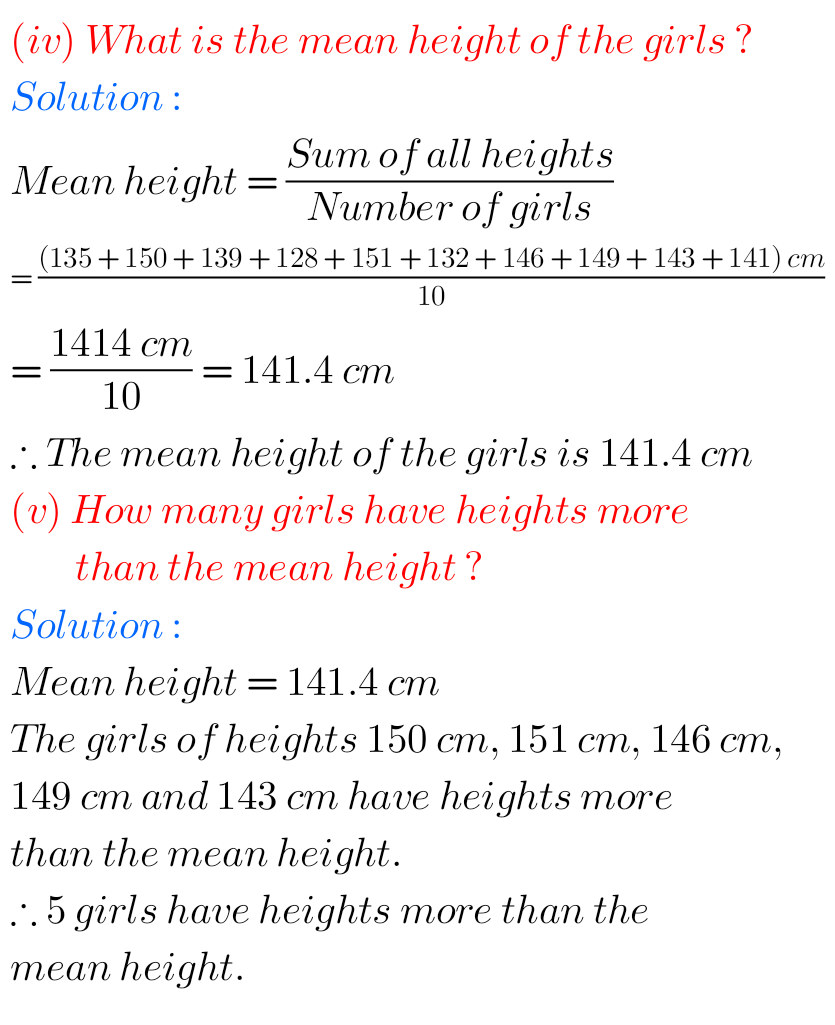# Data Handling exercise 3.1 solutions NCERT maths class 7

NCERT mathematics class 7 Data Handling Chapter 3 exercise 3.1 solutions are given.

You should study the textbook lesson Data Handling very well.

You should also practice all example problems and solutions given in the textbook.

Observe the solutions and try them in your own method.

NCERT Maths class 7th solutions
Data Handling

## Chapter 3 Data Handling exercise 3.1 solutions class 7 maths NCERT

Data Handling

Chapter 3

Exercise 3.1

mmmmmm### Solutions for exercise 3.1 Data Handling class 7 maths NCERT

mmmmmmmm

Note: Observe the solutions and try them in your own method.

Ncert maths solutions for class 7th

NCERT maths solutions for class 6th

SSC maths solutions for class 10th

Inter maths solutions

NIOS Maths 311 book 2 solutions for some chapters